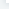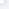# NumPy 线性代数

NumPy 线性代数NumPy 提供了线性代数函数库 linalg，该库包含了线性代数所需的所有功能，可以看看下面的说明：函数描述dot两个数组的点积，即元素对应相乘。vdot 两个向量的点积inner 两个数组的内积matmul 两个数组的矩阵积determinant 数组的行列式solve 求解线性矩阵方程inv 计算矩阵的乘法逆矩阵numpy.dot()numpy.dot() 对于两个一维的数组，计算的是这两个数组对应下标元素的乘积和(数学上称之为内积)；对于二维数组，计算的是两个数组的矩阵乘积；对于多维数组，它的通用计算公式如下，即结果数组中的每个元素都是：数组a的最后一维上的所有元素与数组b的倒数第二位上的所有元素的乘积和：dot(a, b)[i,j,k,m] = sum(a[i,j,:

] * b[k,:

,m])。numpy.dot(a, b, out=None) 参数说明： a :

ndarray 数组 b :

ndarray 数组 out :

ndarray, 可选，用来保存dot()的计算结果实例import numpy.matlibimport numpy as npa = np.array([[1,2],[3,4]])b = np.array([[11,12],[13,14]])print(np.dot(a,b))输出结果为：[[37 40] [85 92]]计算式为：[[1*11+2*13, 1*12+2*14],[3*11+4*13, 3*12+4*14]]numpy.vdot()numpy.vdot() 函数是两个向量的点积。 如果第一个参数是复数，那么它的共轭复数会用于计算。 如果参数是多维数组，它会被展开。实例import numpy as npa = np.array([[1,2],[3,4]])b = np.array([[11,12],[13,14]])

# vdot 将数组展开计算内积print (np.vdot(a,b))输出结果为：130计算式为：1*11 + 2*12 + 3*13 + 4*14 = 130numpy.inner()numpy.inner() 函数返回一维数组的向量内积。对于更高的维度，它返回最后一个轴上的和的乘积。实例import numpy as npprint (np.inner(np.array([1,2,3]),np.array([0,1,0])))

# 等价于 1*0+2*1+3*0输出结果为：2多维数组实例import numpy as npa = np.array([[1,2], [3,4]])print ('数组 a：')print (a)b = np.array([[11, 12], [13, 14]])print ('数组 b：')print (b)print ('内积：')print (np.inner(a,b))输出结果为：数组 a：[[1 2] [3 4]]数组 b：[[11 12] [13 14]]内积：[[35 41] [81 95]]数组 a：[[1 2] [3 4]]数组 b：[[11 12] [13 14]]内积：[[35 41] [81 95]]内积计算式为：1*11+2*12, 1*13+2*14 3*11+4*12, 3*13+4*14numpy.matmulnumpy.matmul 函数返回两个数组的矩阵乘积。 虽然它返回二维数组的正常乘积，但如果任一参数的维数大于2，则将其视为存在于最后两个索引的矩阵的栈，并进行相应广播。另一方面，如果任一参数是一维数组，则通过在其维度上附加 1 来将其提升为矩阵，并在乘法之后被去除。对于二维数组，它就是矩阵乘法：实例import numpy.matlibimport numpy as npa = [[1,0],[0,1]]b = [[4,1],[2,2]]print (np.matmul(a,b))输出结果为：[[4 1] [2 2]]二维和一维运算：实例import numpy.matlibimport numpy as npa = [[1,0],[0,1]]b = [1,2]print (np.matmul(a,b))print (np.matmul(b,a))输出结果为：[1 2] [1 2]维度大于二的数组 ：实例import numpy.matlibimport numpy as npa = np.arange(8).reshape(2,2,2)b = np.arange(4).reshape(2,2)print (np.matmul(a,b))输出结果为：[[[ 2 3] [ 6 11]] [[10 19] [14 27]]]numpy.linalg.det()numpy.linalg.det() 函数计算输入矩阵的行列式。行列式在线性代数中是非常有用的值。 它从方阵的对角元素计算。 对于 2×2 矩阵，它是左上和右下元素的乘积与其他两个的乘积的差。换句话说，对于矩阵[[a，b]，[c，d]]，行列式计算为 ad-bc。 较大的方阵被认为是 2×2 矩阵的组合。实例import numpy as npa = np.array([[1,2], [3,4]])print (np.linalg.det(a))输出结果为：-2.0实例import numpy as npb = np.array([[6,1,1], [4, -2, 5], [2,8,7]])print (b)print (np.linalg.det(b))print (6*(-2*7 - 5*8) - 1*(4*7 - 5*2) + 1*(4*8 - -2*2))输出结果为：[[ 6 1 1] [ 4 -2 5] [ 2 8 7]]-306.0-306numpy.linalg.solve()numpy.linalg.solve() 函数给出了矩阵形式的线性方程的解。考虑以下线性方程：x + y + z = 62y + 5z = -42x + 5y - z = 27可以使用矩阵表示为：如果矩阵成为A、X和B，方程变为：AX = B或X = A^(-1)Bnumpy.linalg.inv()numpy.linalg.inv() 函数计算矩阵的乘法逆矩阵。逆矩阵（inverse matrix）：设A是数域上的一个n阶矩阵，若在相同数域上存在另一个n阶矩阵B，使得： AB=BA=E ，则我们称B是A的逆矩阵，而A则被称为可逆矩阵。注：E为单位矩阵。实例import numpy as npx = np.array([[1,2],[3,4]])y = np.linalg.inv(x)print (x)print (y)print (np.dot(x,y))输出结果为：[[1 2] [3 4]][[-2. 1. ] [ 1.5 -0.5]][[1.0000000e+00 0.0000000e+00] [8.8817842e-16 1.0000000e+00]] 现在创建一个矩阵A的逆矩阵:

# 这就是线性方向 x = 5, y = 3, z = -2 的解输出结果为：数组 a：[[ 1 1 1] [ 0 2 5] [ 2 5 -1]]a 的逆：[[ 1.28571429 -0.28571429 -0.14285714] [-0.47619048 0.14285714 0.23809524] [ 0.19047619 0.14285714 -0.0952381 ]]矩阵 b：[[ 6] [-4] ]计算：A^(-1)B：[[ 5.] [ 3.] [-2.]]结果也可以使用以下函数获取：x = np.dot(ainv,b)

NumPy 线性代数

## 相关文章